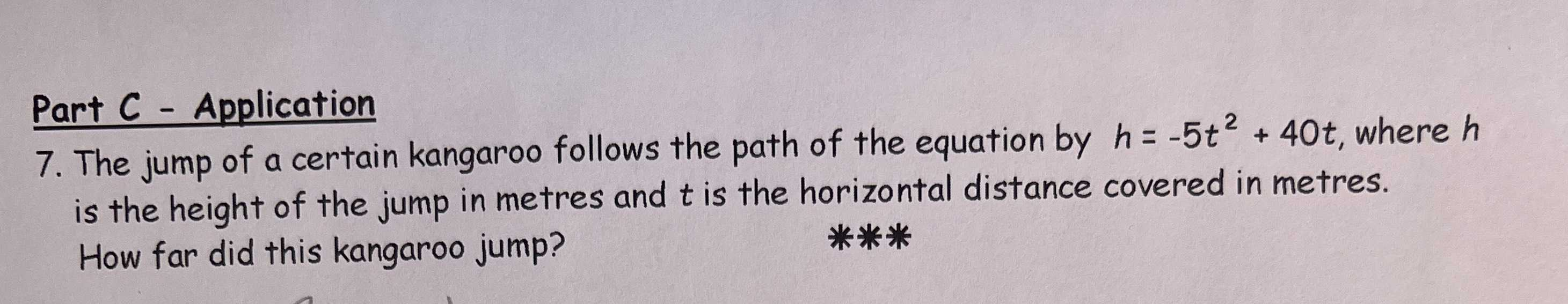### ¿Todavía tienes preguntas de matemáticas?

Pregunte a nuestros tutores expertos
Algebra
Pregunta7. The jump of a certain kangaroo follows the path of the equation by $$h = - 5 t ^ { 2 } + 40 t$$ , where $$h$$ is the height of the jump in metres and $$t$$ is the horizontal distance covered in metres. How far did this kangaroo jump?

$$( 4,80 )$$